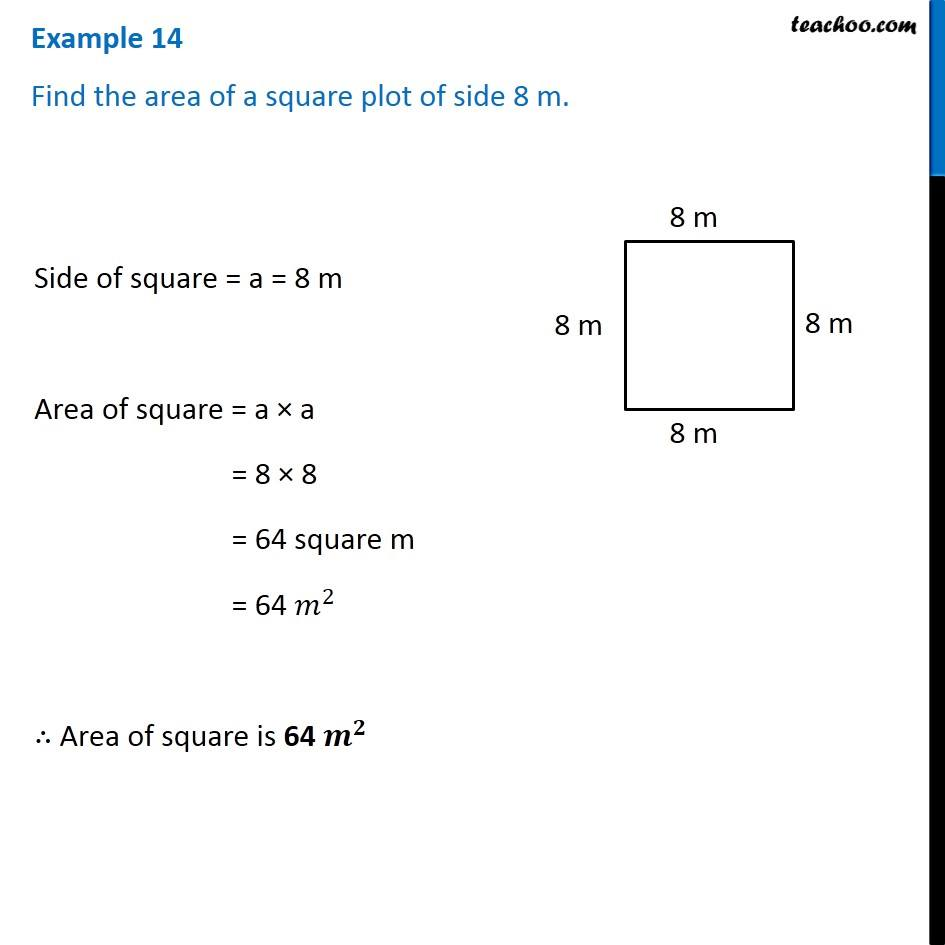Examples

Chapter 10 Class 6 Mensuration
Serial order wiseLearn in your speed, with individual attention - Teachoo Maths 1-on-1 Class

### Transcript

Example 14 Find the area of a square plot of side 8 m. Side of square = a = 8 m Area of square = a × a = 8 × 8 = 64 square m = 64 𝑚^2 ∴ Area of square is 64 𝒎^𝟐